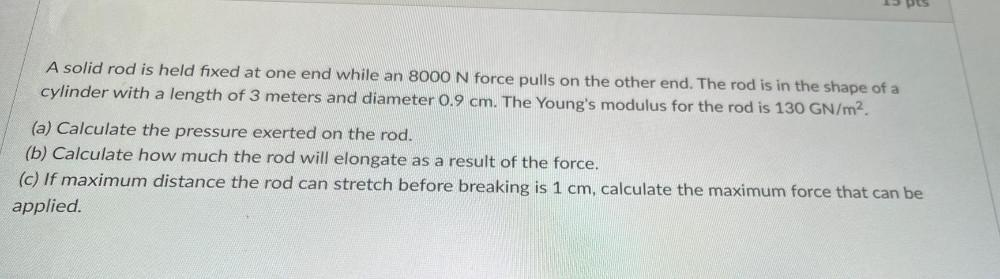Question:

# A solid rod is held fixed at one end while an 8000 N force pulls on the other end. The rod is in the shape of a cylinder with a length of 3 meters and diameter 0.9 cm. The Young's modulus for the rodA solid rod is held fixed at one end while an 8000 N force pulls on the other end. The rod is in the shape of a cylinder with a length of 3 meters and diameter 0.9 cm. The Young's modulus for the rod is 130 GN/m². (a) Calculate the pressure exerted on the rod. (b) Calculate how much the rod will elongate as a result of the force. (c) If maximum distance the rod can stretch before breaking is 1 cm, calculate the maximum force that can be applied.